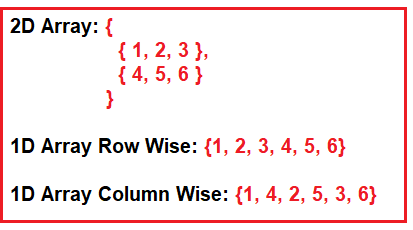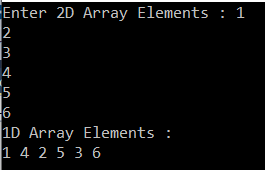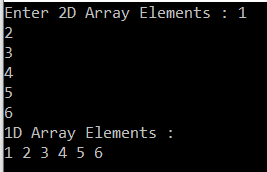# How to convert a two-dimensional array to one-dimensional array in C#

## How to convert a two-dimensional array to one-dimensional array in C#?

In this article, I am going to discuss how to convert a two-dimensional array to one-dimensional array in C# with some examples. Please read our previous article where we discussed How to Find All Possible Substring of a Given String in C# with some examples. As part of this article, we will discuss the following two approaches.

1. How to convert a 2d array into 1d array column-wise in C#?
2. How to convert a 2d array into 1d array row-wise in C#?
##### Program Description:

Here, the user will input a two-dimensional array (i.e. matrix) and we need to convert that 2D array to a one-dimensional array. Here, we will create the one-dimensional array row-wise as well as column-wise. For better understanding please have a look at the following diagram.##### Creating a 1D Array from 2D Array Column Wise in C#:

The following program is self-explained. So, please go through the comment lines for better understanding. In the following example, we convert the 2d array to 1d array column-wise.

```using System;
namespace LogicalPrograms
{
class Program
{
static void Main(string[] args)
{
//Creating a 2d Array with 2 rows and three columns
int[,] int2DArray = new int[2, 3];
Console.Write("Enter 2D Array Elements : ");
for (int i = 0; i < 2; i++)
{
for (int j = 0; j < 3; j++)
{
}
}

int index = 0;

//Getting the no of rows of 2d array
int NoOfRows = int2DArray.GetLength(0);

//Getting the no of columns of the 2d array
int NoOfColumns = int2DArray.GetLength(1);

//Creating 1d Array by multiplying NoOfRows and NoOfColumns
int[] OneDimensionalArray = new int[NoOfRows * NoOfColumns];

//Assigning the elements to 1d Array from 2d array
for (int y = 0; y < NoOfColumns; y++)
{
for (int x = 0; x < NoOfRows ; x++)
{
OneDimensionalArray[index] = int2DArray[x, y];
index++;
}
}

//Printing the 1d array elements
Console.WriteLine("1D Array Elements : ");
foreach (int item in OneDimensionalArray)
{
Console.Write(item + " ");
}

}
}
}
```

Output:##### Creating the 1D Array From 2D Array Row Wise in C#:

In the following program, we convert the two-dimensional array to one-dimensional row-wise. For better understanding please go through the comment lines.

```using System.Linq;
using System;

namespace LogicalPrograms
{
class Program
{
static void Main(string[] args)
{
//Creating a 2d Array with 2 rows and three columns
int[,] int2DArray = new int[2, 3];
Console.Write("Enter 2D Array Elements : ");
for (int i = 0; i < 2; i++)
{
for (int j = 0; j < 3; j++)
{
}
}

int index = 0;

//Getting the no of rows of 2d array
int NoOfRows = int2DArray.GetLength(0);

//Getting the no of columns of the 2d array
int NoOfColumns = int2DArray.GetLength(1);

//Creating 1d Array by multiplying NoOfRows and NoOfColumns
int[] OneDimensionalArray = new int[NoOfRows * NoOfColumns];

//Assigning the elements to 1d Array from 2d array
for (int y = 0; y < NoOfRows ; y++)
{
for (int x = 0; x < NoOfColumns; x++)
{
OneDimensionalArray[index] = int2DArray[y, x];
index++;
}
}

//Printing the 1d array elements
Console.WriteLine("1D Array Elements : ");
foreach (int item in OneDimensionalArray)
{
Console.Write(item + " ");
}

}
}
}```

Output:In the next article, I am going to discuss how to convert a one-dimensional array to a two-dimensional array in C# with examples. I hope now you understood how to convert a two-dimensional array to one-dimensional array in C# with different mechanisms.

### 1 thought on “How to convert a two-dimensional array to one-dimensional array in C#”

1.hello ? anyone know about about how convert two dimensional grey image g(i, j) to one dimensional array g(k)